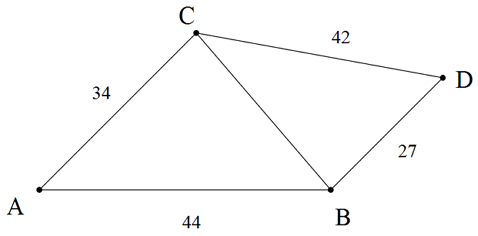# Area Of Quadrilateral Given All Its Sides?

Geometry Level 2Consider a quadrilateral $ABCD$ with sides $AB=44, AC=34, CD=42$ and $BD=27$.
Find the area of the quadrilateral $ABDC$.